# ISEE Middle Level Math : How to add fractions

## Example Questions

### Example Question #441 : Concepts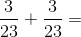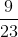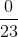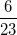Explanation:

Add the numerators and leave the denominators alone: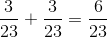Answer:### Example Question #442 : Concepts

Subtract: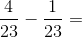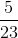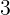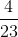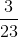Explanation:

Subtract the numerators and leave the denominators alone: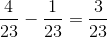Answer:### Example Question #443 : Concepts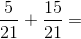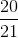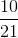Explanation:

Because the two fractions have the same denominator, simply add the numerators and leave the denominator as is: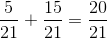Answer:### Example Question #51 : How To Add Fractions

Which of the following statements demonstrates the commutative property of addition?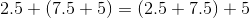None of the examples in the other responses demonstrates the commutative property of addition.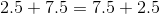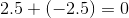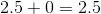Explanation:

The commutative property of addition states that two numbers can be added in either order to obtain the same sum. Of the given responses, onlydemonstrates this property, so it is the correct choice.

### Example Question #444 : Concepts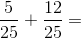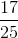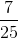Explanation:

Add the numerators and leave the denominator the same: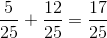Answer:### Example Question #445 : Concepts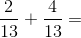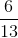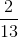Explanation:

Add the numerators and leave the denominator the same: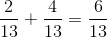Answer:### Example Question #52 : How To Add Fractions

The time is now 10:38 PM. What time will it be in two hours and fifty-two minutes?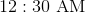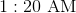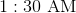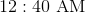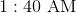Explanation:

It is now 10:38 PM. In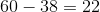minutes, it will be 11:00 PM; in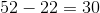minutes, the minutes will read 30, so the time will be 11:30 PM. In another two hours, the hours will read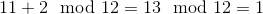,

so the time will be 1:30 AM.

### Example Question #53 : How To Add Fractions

Solve the expression.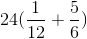None of theseExplanation:

Start by solving for the term in parenthesis. We will need to find the least common denominator in order to add the fractions. Since 6 can be multiplied by 2 to get 12, 12 must be the least common denominator.

Convert the fractions so that they share a denominator of 12.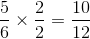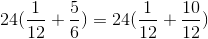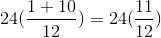Multiply.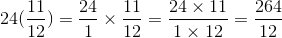You can then simplify the fraction be removing 12 from both the numerator and denominator.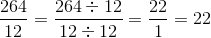### Example Question #54 : How To Add Fractions

Which of the following is the sum of five-sevenths and one-half?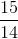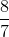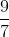The correct answer is not among the other responses.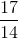Explanation:

Since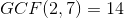, express each fraction as its equivalent in fourteenths, and add the numerators, as follows: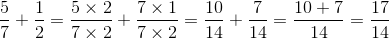### Example Question #55 : How To Add Fractions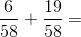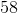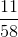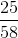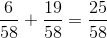Answer: